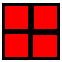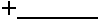Problem Solving 2

Problem Solving Lessons & Tests - My Schoolhouse - Online Learning

 A AA AAA AAAA AAAAA AAAAAA AAAAAAAA AAAAAAA AAAAAA AAAAA AAAA AAA AA A AAAAAAAA AAAAAAA AAAAAA AAAAA AAAA AAA AA A

How many times does the letter A appear in the diagram above?

times.

The square below is divided into four congruent parts.  Each part has a perimeter of 16 units.  What is the perimeter of the square?

units.In the addition problem below, each letter represents a number.  Solve for each letter.

A =

B =

C =

 3 4 2 A BC 3 1

If a number is divided by 3 or 5, the remainder is 1.  If it is divided by 7, there is no remainder.  What number between 1 and 100 satisfies the above condition?

Number

If all the counting numbers were arranged in the chart below, in what letter column would 50 appear?

Column

 A B C D 1 2 3 4 5 6 7 8

 Elementary & Middle School Lessons & Self-Correcting Worksheets for Children in all Subject Areas. Lessons & Worksheets in Math, Reading, Spelling, Science, Language, and Social Studies.     My Schoolhouse Copyright 1999-2019 by Educational Designers, LLC. All rights reserved.